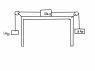# Tension problem, finding acceleration with kinetic friction

• p0ps1c1e

#### p0ps1c1e

Three blocks are connected as shown in the uploaded picture. The strings and friction-less pulleys have negligible masses, and the coefficient of kinetic friction between the 2.0 kg block and the table is 0.17. What is the acceleration of the 2.0 kg block?

∑F = ma

## The Attempt at a Solution

So i wrote 3 Newton equations:

k-T1+T2 = m2a

T1-m1g = m1a

T2-m3g = m3a

Putting them together I got:

km2g - m1g + m3g = a(m2 + m1 - m3)

and then tried to solve for acceleration. Problem is every time I do it I end up with a 0 in the denominator so I don't know if I'm doing something wrong conceptually or if I am just messing up in my algebra. I'm also not sure if all three of the blocks have the same acceleration. I would think so since they are all on a string.

#### Attachments

•PhysicsDrawing1.png
18.3 KB · Views: 602
Last edited:
Your equation do not match your drawing... but I think I can figure it out.
m1=1kg, m2=2kg and m3=3kg right?

The first equation is incorrect - what is the equation governing friction?

The last equation is inconsistent with the first two - check the direction for positive acceleration.

Oh derp... haha. Thanks.

I'm looking through my old exams and I'm finding so many little mistakes like that.